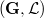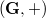﻿

### A characterization of the affine Hall triple systems defined by groups of exponent 3

#### Abstract

In this paper we show that if$(\mathbf{G}, \mathcal{L})$ is the Hall      triple system associated with a group of exponent 3$(\mathbf{G}, +)$,      then$(\mathbf{G}, \mathcal{L})$ is an affine space if and only if$(\mathbf{G}, +)$ is nilpotent of class at most 2.

DOI Code: 10.1285/i15900932v30n2p97

Keywords: affine Hall triple systems; groups of exponent 3

Full Text: PDF